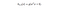# The Math Behind SVM

Part 1: Implementing SVM from scratch

website: https://eliteaihub.com/

SVM or Support Vector Machine is a linear model for classification and regression problems. It can solve linear and non-linear problems and work well for many practical problems. The idea of SVM is simple: The algorithm creates a line or a hyperplane which separates the data into classes.

The goal of SVM is to identify an optimal separating hyperplane which maximises the margin between different classes of the training data.

We will be considering a linear classifier for a binary classification problem with labels y and features x.

# Know the problem?

consider the following figure, in which blue ‘o’ represent positive training examples, green ‘o’s denote negative training examples, a decision boundary (this is the line given by the equation θ^ T* x = 0, and is also called the separating hyperplane) is also shown.each have labels of either y=+1 or y= -1 class, and our examples are linearly separable. Then, our training data is form <Xi, Yi>

The dataset above is linearly separable, hence we can formulated as,

Thus, Hypothesis Function for SVM can be defined as:w, b are parameters, x is the features used for the model

The goal of SVM is to identify an optimal separating hyperplane which maximises the margin between different classes of the training data. i.e., we want to maximise γ, subject to each training example having functional margin at least γ,

Given a training set

S = {(x (i) , y (i) );i = 1, . . . , m}, we also define the function margin of (w, b) with respect to S as the smallest of the functional margins of the individual training examples. Denoted by γ(i). Hence, a large functional margin represents a confident and a correct prediction.

H1: Positive hyperplane,

H2: Negative Hyperplane

H1 = { w.x + b = 1 } H2 = { w.x + b = -1}

γ = (1 -b)/|w| — (-1-b)/|w| = 2/|w|

thus margin γ1 and γ2 should be γ/2 = 1/|w|

Thus, maximizing 1/|w| is same equivalent as,

## Solving a Quadratic programming problem?

Problem is: minimise ||w||, s.t. discrimination boundary is obeyed, i.e., min f(x) s.t. g(x)=0, which we can rewrite as: min f: s.t. g: yi (w•xi )–b = 1 or [yi (w•xi )–b] — 1 =0.

1/2||w||² (Note this is a quadratic function) This is a constrained optimisation problem, with constraint , yi (w•xi )–b = 1 for this linearly separable binary problem in hand.

Can we use Gradient Descent for Optimisation?

SVM optimisation problem is a case of constrained optimisation problem, and it is always preferred to use dual optimisation algorithm to solve such constrained optimisation problem. In fact, The hinge loss is not a differentiable function! hence, gradient descent is not preferred.

Since it is constrained optimisation problem Lagrange multipliers are used to solve it!

Thanks for reading. Stay tuned for next story — Part2: Lagrange multipliers, and much more math behind SVM’s

Helping you build something Innovative…

## More from EliteAI

Helping you build something Innovative…